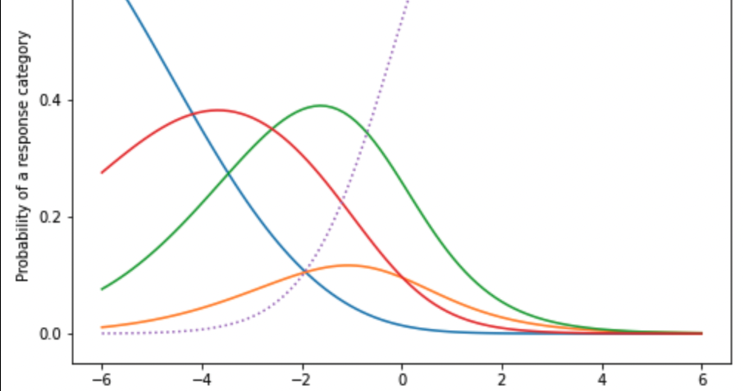The goal of a Triplebyte assessment is to allow engineers to demonstrate their true skill levels and help employers identify skilled engineers to reach out to. When we only have a short quiz to achieve this, we need to extract as much data as we can out of the information that we have.

Multicategorical Item Response Theory (IRT) models help us do this. (See our introduction on IRT we published a few years ago.) In simpler IRT models, responses are categorized as correct or incorrect, and we model the probability of getting a correct response as a function of underlying skill. In multicategorical IRT models, responses have more than two categories, and they correspond to varying levels of incorrectness. We model the probabilities of the response being in each category as a function of skill. These probability curves are illustrated below. In the first graph, the question is scored as correct or incorrect. In the second graph, the same question is scored in five categories.

In the graph above, as skill increases past 0 (in standard units), the probability of getting this question correct surpasses the probability of getting this question incorrect, and as skill decreases past 0, the probability of getting this question incorrect surpasses the probability of getting it correct. Therefore, the incorrect response is the most probable for test-takers with skill below 0, and the correct response is the most probable for test-takers with skill above 0.

In this second graph, response category 1 (blue) is the most probable response category for test-takers with skill below -4 (approximately), response category 4 (red) is the mostly probable for test-takers with skill between -4 and -3, response category 3 (green) is the most probable for test-takers with skill between -3 and -1, and the correct response (dotted purple) is the most probable for test-takers with skill above -1. The additional categories shown in the right graph add granularity to our scoring scale, which ultimately translates to more precision in our score estimates.

For certain types of assessments, we are fairly confident we know the rank order of the categories a priori. For our timed coding questions, for example, we assume that, for a given solution, passing some test cases requires more skill than passing no test cases, and passing all test cases requires more skill than passing some test cases. Therefore, we can categorize a response into one of three ordered categories. Hypothetically, we could also have human graders assign grades of 0, 1, or 2, for example, to engineer responses, and these grades would be ordered into response categories where a higher grade is better than a lower grade.

For other assessment types, it is not obvious what the rank order of the categories should be, so we use a model to determine that empirically. This is the case with our multiple-choice questions. We can consider the selection of each answer option a response category. We don’t know a priori the rank order of selecting the distractors, or wrong answer options, but our model can estimate that for us.

Obtaining the empirical rank order of the answer options is also useful for the question development process. If the rank order we discover through the model for a given question is not what we expected, it suggests that the question might be a bad question, thereby identifying an opportunity for question quality improvement. In the graph below, as skill increases past 1, the probability of selecting the correct answer option (dotted purple), becomes lower than the probability of selecting one of the distractors (red). This is unexpected and undesired, since we expect the probability of answering each question correctly to increase as skill increases. If this question has also been flagged by other statistics as problematic, then we can feel pretty confident in removing or replacing it.

In addition to monitoring question quality, we can also monitor the quality of our distractors. To get the most data out of each question, we want each distractor to contribute additional information. Otherwise, we’re wasting opportunities to get more data. In the graph below, response category 2 (yellow) is never the most probable at any point in the skill range, which suggests we can do just as well by not having it. Knowing this, our question writers can then try to replace it with something that does provide more information.

Using multicategorical models, we get more out of the data we already have. We increase the precision of our scores without making engineers spend more time answering questions. Companies have better data to make decisions on whom to reach out to, thus saving time in their hiring process. Meanwhile, having insights into the contribution of each response category allows us to constantly improve our questions. All of this, we believe, make our tests even more fair and efficient.